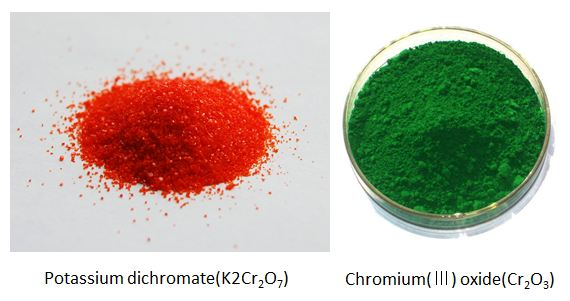# Redox reaction

Chemistry Level 1Redox (reduction-oxidation) reactions include all chemical reactions in which atoms have their oxidation state changed; in general, redox reactions involve the transfer of electrons between species. The above left figure shows potassium dichromate and the above right one depicts chromium (III) oxide. The reaction formula for creating chromium (III) oxide from potassium dichromate is as follows: \begin{aligned} \text{2K}_2\text{Cr}_2\text{O}_7(aq) &+2\text{H}_2\text{O}(l)+\text{3S}(s) \\ &\to \text{4KOH}(aq)+\text{2Cr}_2\text{O}_3(s)+\text{3SO}_2(g). \end{aligned} What are the oxidizing agent and the reducing agent?

×